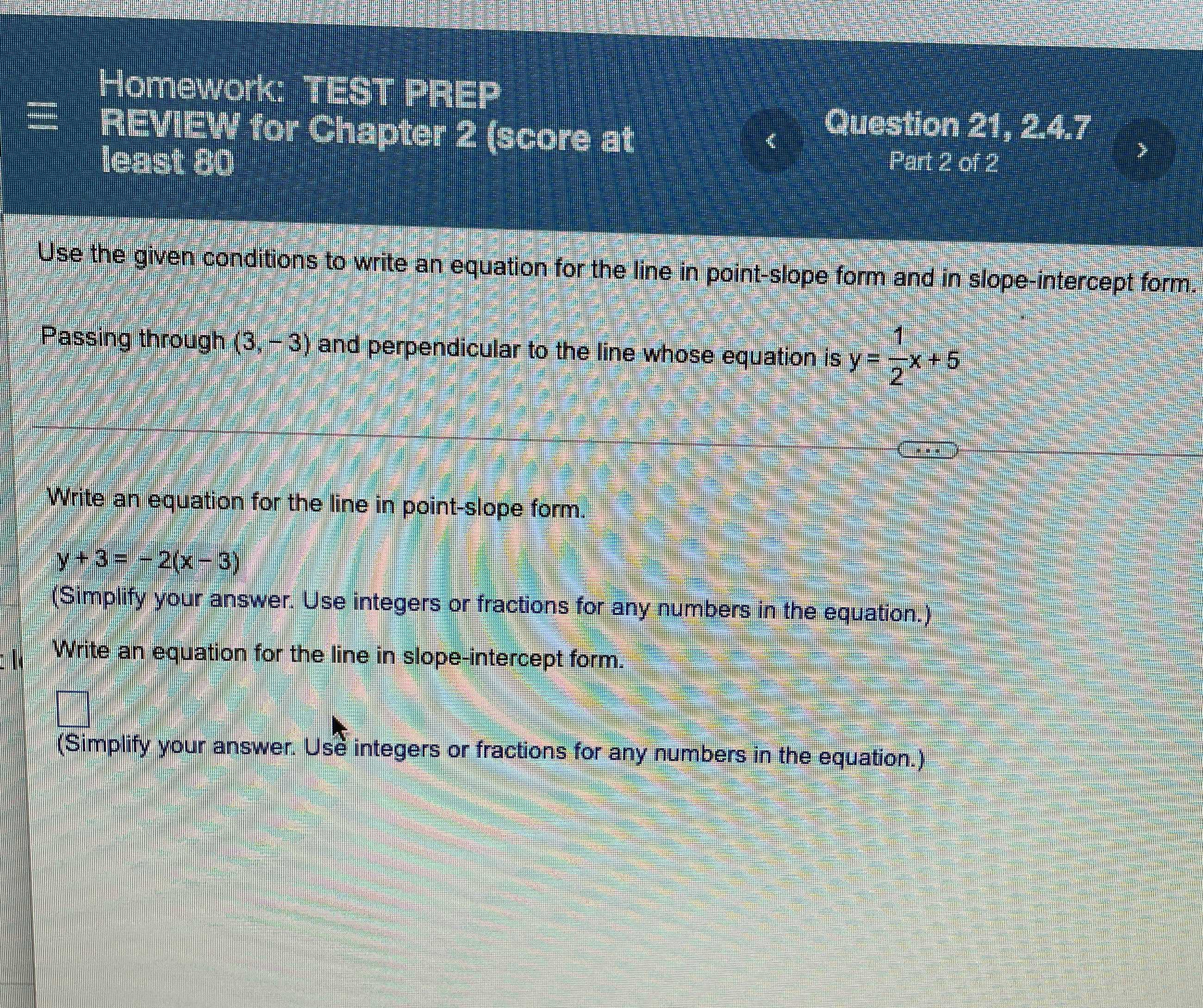### Still have math questions?

Algebra
QuestionUse the given conditions to write an equation for the line in point-slope form and in slope-intercept form. Passing through $$( 3 , - 3 )$$ and perpendicular to the line whose equation is $$y = \frac { 1 } { 2 } x + 5$$

Write an equation for the line in point-slope form.

$$y + 3 = - 2 ( x - 3 )$$

(Simplify your answer. Use integers or fractions for any numbers in the equation.) Write an equation for the line in slope-intercept form. (Simplify your answer. Use integers or fractions for any numbers in the equation.)